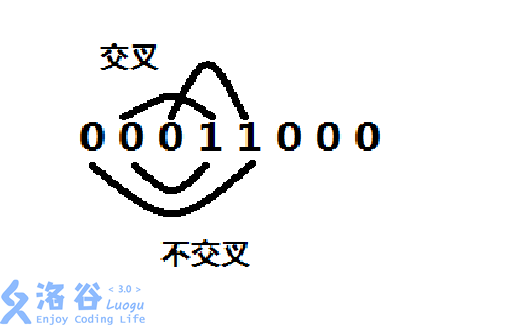# P4989 二进制之谜

• 19通过
• 177提交
• 题目提供者 Forward_Star
• 评测方式 云端评测
• 标签 洛谷原创
• 难度 提高+/省选-
• 时空限制 2000ms / 256MB
• 提示：收藏到任务计划后，可在首页查看。

## 题目背景

虽然过了$Faded$，但是小埋还是没有解出二进制之谜。

## 题目描述

这个时候，她感觉到了$0$与$1$存在的某种可能的特殊对应关系。于是，她定义了“启发系数”：对应的两个数位数（按从高位到低位顺序去数）的差的绝对值；她现在希望将$0$与$1$进行对应使得在对应关系最多的前提下，启发系数之和最大。

对应规则如下：

$1$.对应关系必须从$0$开始，以$1$结束；换句话说，每个对应关系必须$0$在前（高位），$1$在后（低位）；

$2$.可以取若干个对应关系，但对应关系之间不能交叉交叉的含义是：共用某个区间且不是包含关系；

$e.g.$ 假设一个对应关系为第$2$位数与第$4$位数，另一个对应关系为第$3$位数与第$5$位数，那么它们不可同时取，因为在区间$[3,4]$交叉；但是若对应关系分别为第$1$、$5$位数与第$2$、$4$位数，则不算作交叉，因为它们虽然共用区间$[2,4]$但存在包含关系，可以同时取。这即是说，交叉不等于交集

$3$.每个数最多只能存在于一个对应关系中。

## 输入输出格式

输入格式：

第一行，一个整数$n$，为二进制数的位数；

接下来一行，输入一个$n$位二进制数。

输出格式：

一行，表示对应关系最多的前提下最大的启发系数之和。

## 输入输出样例

输入样例#1： 复制
2
10
输出样例#1： 复制
0
输入样例#2： 复制
6
110100
输出样例#2： 复制
1

## 说明

对于$30$%的数据，$0<n<=20$；

对于$100$%的数据，$0<n<=300$；

样例说明

对于样例一，由于$1$在$0$前面，两者不能对应；

对于样例二，对应方案为$3-4$，故总和为$1$。

如果您提前$AK$了，可以做一下数据增强版

提示
标程仅供做题后或实在无思路时参考。
请自觉、自律地使用该功能并请对自己的学习负责。
如果发现恶意抄袭标程，将按照I类违反进行处理。